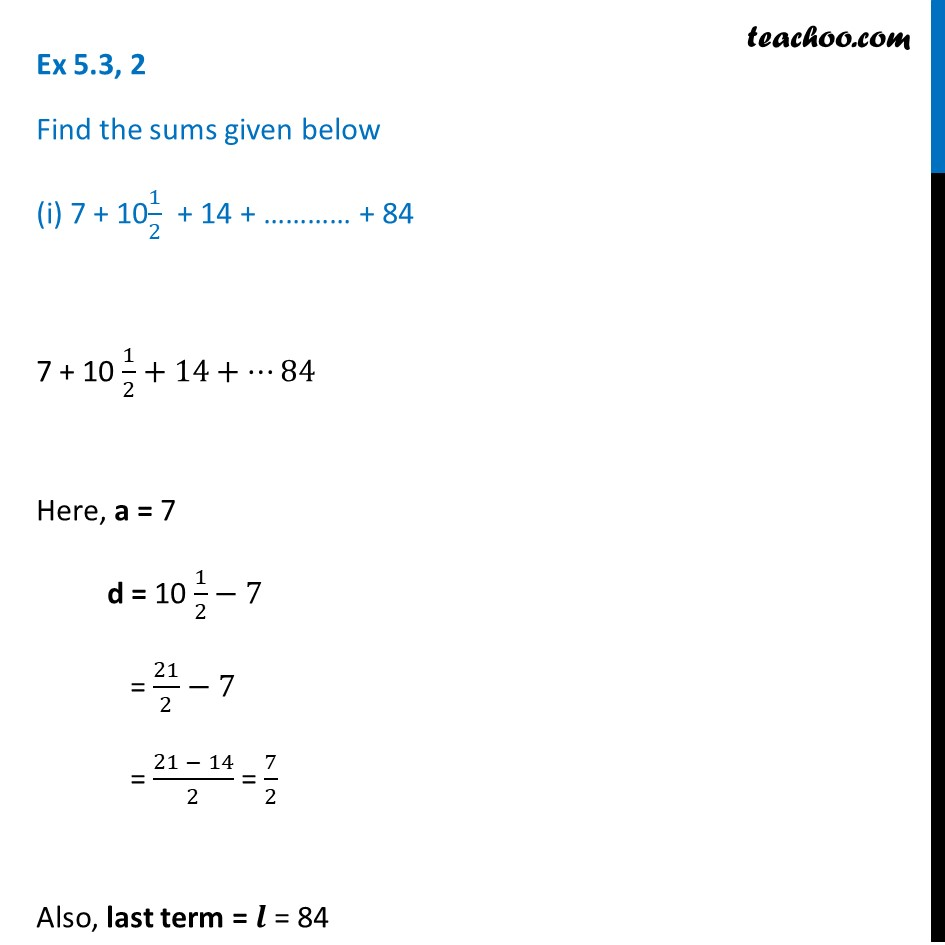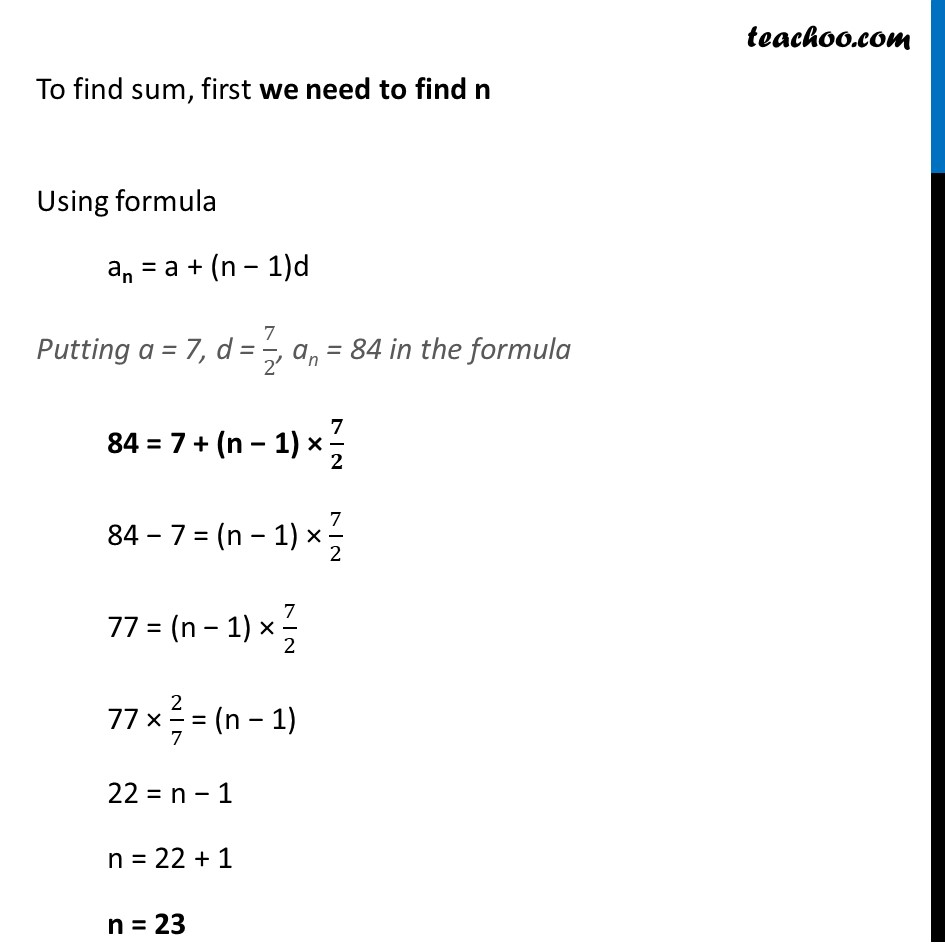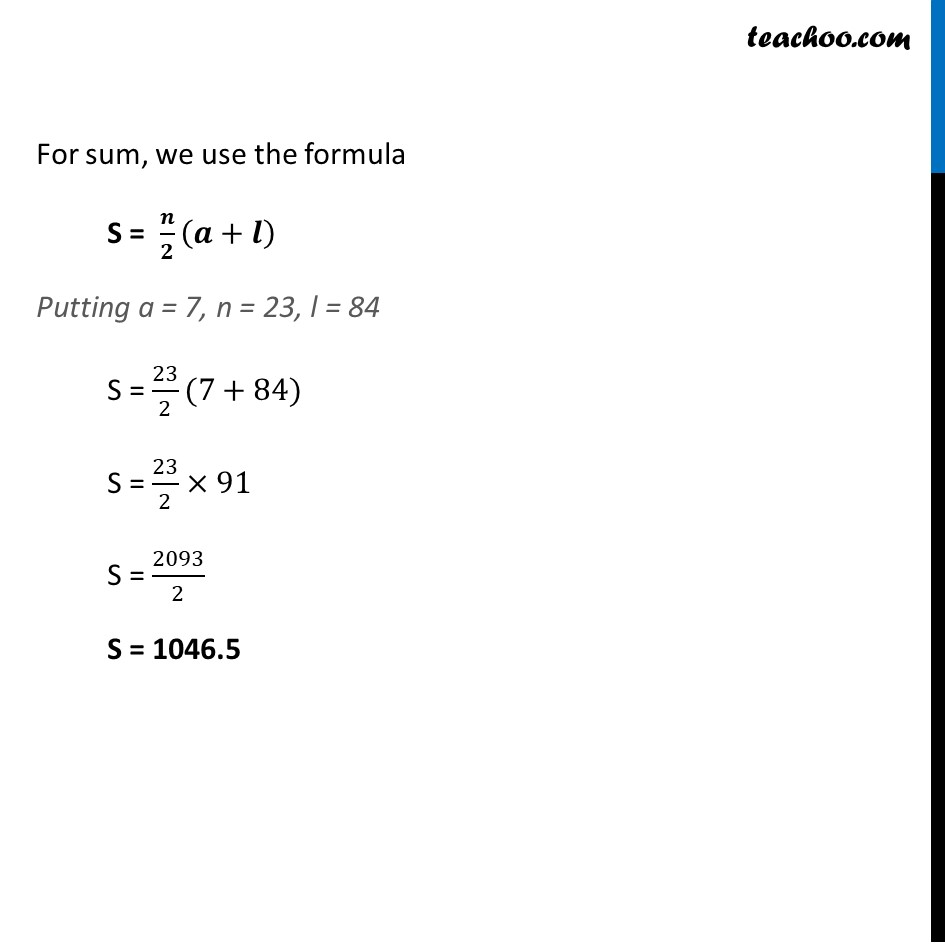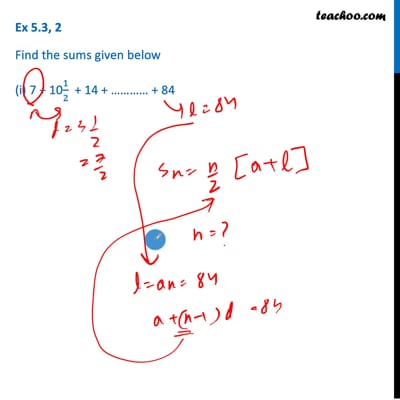Finding sum of n terms

Chapter 5 Class 10 Arithmetic Progressions (Term 2)
Concept wiseThis video is only available for Teachoo black users

### Transcript

Ex 5.3, 2 Find the sums given below (i) 7 + 101/2 + 14 + ………… + 84 7 + 10 1/2+14+…84 Here, a = 7 d = 10 1/2−7 = 21/2−7 = (21 − 14)/2 = 7/2 Also, last term = 𝒍 = 84 To find sum, first we need to find n Using formula an = a + (n − 1)d Putting a = 7, d = 7/2, an = 84 in the formula 84 = 7 + (n − 1) × 𝟕/𝟐 84 − 7 = (n − 1) × 7/2 77 = (n − 1) × 7/2 77 × 2/7 = (n − 1) 22 = n − 1 n = 22 + 1 n = 23 For sum, we use the formula S = 𝒏/𝟐 (𝒂+𝒍) Putting a = 7, n = 23, l = 84 S = 23/2(7+84) S = 23/2×91 S = 2093/2 S = 1046.5